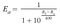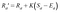# Implementing the Elo Rating System

In this article we’ll look at how to implement the Elo rating system in code.

This is part of a series of articles on the Elo Rating system.

1. We developed an understanding of the Elo rating system and walked through the equations with interactive visuals.
2. (Current) Create a rating system library in TypeScript
3. We look closer at the simulation tool and talk about about some of the more advanced adaptations you can make when applying in different contexts.

I’ll be writing TypeScript since I plan to consume it as an NPM package in other TypeScript projects but as you can imagine in the first article the core of code is mostly writing math equations and could be done in any language you prefer. We’ll look at two different projects:

1. The actual rating system package which implements the Elo system
This would be distributed and used by other applications.
2. A tool to simulate games using the package above.
This helps verify expected operation over a larger scale than the unit tests.

# The Rating SystemExpected probability for player A given rating of player A (Ra) and rating of player B (Rb)Next Rating for Player A given current rating for Player A, K-factor, Score and expected probability above

We see the variables of the rating system which can be adjusted.

## 2. Scale Factor (Seen as constant 400)

For example, it is preferable and easier to understand a player with rating 2400 is substantially better than a player with rating of 1000. Where as if the scale factor was left at 1, the same skills difference would be between players with ratings 4.5 and 1. Both systems would produce an exponent value of 3.5.(2400–1000)/400 = 4.5–1. However, in the latter system a seemingly insignificant decimal difference of players ratings we would actually mean a significant skills difference and this mismatch makes it less intuitive. However, in the former system the the meaningful skills differences would be in the hundreds which would is on a similar scale to other meaningful differences of day to day numbers humans experience.

## Create The System

`export function createRatingSystem(kFactor: KFactorOption = 32, exponentDenominator = 400, exponentBase = 10): RatingSystem`

## Compute the Probabilities

Notice: we use these “create*” prefixed functions because we’re using currying for deferred execution. We don’t want to compute the probabilities and ratings immediately but product a system that will compute them given the appropriate k-factor, scale-factor, etc.

Remember, this function takes a rating difference. We now have to supply it the appropriate differences given the player probabilities to create the full expected probability equation.

Notice: We use the `expectedPlayerProbability` function twice, once for each player, then return all necessary data. In most applications you would only need the first two inputs, but we return the rating differences as extra information for consumer so they don’t have to compute it, while avoiding adding noise to the API.

## Computing The Next Rating

This equation takes in the current player rating, the score, and expected score which is the computed probability.

Note: In the simple case the K Factor is just a number such as 32, but in more advanced cases it decreases as the player rating increase to align with the increased stability in play as they become more experienced.

Notice similar to the expected probabilities function, we return the `nextRating` and also the `change` in rating so the consumers don’t have to compute it.

## Putting it Together

We could stop here. Users could consume our library and have a working rating system using an example such as this:

This works but exposes an amount of standard and repetitive work for the consumer that could easily be absorbed. There are 2 main points:

1. Having to compute both scores. Knowing one score is sufficient to know the other so it’s unnecessary explicitly provide both
2. Having to pass correctly aligned arguments when getting the next ratings. It would be easy to mistakenly pass the wrong rating or the wrong score when computing the ratings and this could also be internalized without sacrificing data

## Building A Nicer API

Instead of exposing two functions, we could expose a single `getNextRatings` function that takes in player a’s rating, players b’s rating, and the score of the game and computes the next ratings for both players outputting all the same information we computed above.

This would be used a a single line as seen below:

Ah. much nicer. 🙌

## Rating Dependent K-Factor

We know the create function can take a k factor that’s a function or a number. If a number is given we just create a function that returns that number so they are both functions.

Instead of simply performing `K * (S — E)` as seen in the equation, we use the function and give it the rating of the current player. Example: `kFactorFn(rating) * (actualScore — expectedScore)`

As you may have read from the the Wiki:

https://en.wikipedia.org/wiki/Elo_rating_system#Most_accurate_K-factor

Players below 2100: K-factor of 32 used

Players between 2100 and 2400: K-factor of 24 used

Players above 2400: K-factor of 16 used.

Because we use a function which is given the rating we could dynamically change our K Factor if we wanted.

## Player Dependent K-Factor

That’s a great question and shows you have some intuition as to what player dependent K Factors would mean in your system. As you may have read, these rating systems are normally used for zero-sum games. This means if one player gains rating (+n) another player must lose equal rating (-n). (Side Note: This kind of reminds me of the Law of Conservation of Energy)

From what I’ve read I haven’t seen this player dependent technique used or mentioned so it’s a bit experimental, but that’s what makes it new and exciting. In my limited testing and understanding it works as expected without major penalties. My concerns would be with even more advanced issues in these systems such as “rating inflation” or “rating drift” which you can read about from the resources linked in the first article. From my understand rating inflation is similar to money inflation. Say rating 1000 means X skill today, but after some time the equivalent X amount of skill is now rated as 1500. Another complication is rating drift where ratings increase or decrease over time due to large changes in player population and other longer term effects.

We’ll look more at testing in the next article. I wouldn’t consider my simulator testing extensive but it is reassuring that you can observe many iterations and see all the changes to players.

## Back to the Question

In my particular case, I was developing a rating system for a game where it’s not a typical game of two humans players competing against each other. Rather one player is a human and the other “player” is a Quiz question. As the player answers questions correctly he effectively wins and his rating goes up indicating he is more knowledgeable. I also wanted the questions to self adjust to their appropriate skill rating. However, since they are not humans and cant gain experience and learn they shouldn’t be treated the same.

We want the players to have normal K Factor, but for the questions they will be created and initialized to a hopefully accurate rating and should be more stable and resistant to change. If a question is going to drop rating it should likely take a sizeable influence. Say a larger number of players who were expected to answer the question correctly got it wrong, then perhaps that question should be rated lower to be more fair and be better representative for players of that level.

Now that we understand the rational for player dependent K-Factors lets look at implementation.

Implementing Player Depending K-Factors

Recall our current system takes in a K Factor function or a number:
`type KFactorFunction = (rating: number) => number`
`kFactor: number | KFactorFunction`

but we want a signature which includes the player as input. It could be a player A or player B (which in our case we know is a Question). We could use enums: “a” | “b”, but I chose to keep numbers since almost everything in this library is a number. It is treated like a index. Player A is index 0 and Player B is index 1.

`type KFactorFunctionWithPlayers = (rating: number, playerIndex: number | undefined) => number`

Recall in our `getNextRatings` functions we’re in control of passing the K factor to the rating function. This function is created in our `createRatingSystem` function. It is there we can then create these K-Factor functions with players.

Here is the final form of the create rating system which accepts 3 types for K. Two value which would produce a symmetric system a number or function only concerned with player rating, or they can give a function that relies on player index to potentially produce what I’m calling asymmetric system since players aren’t treated equally.

You can see for the symmetric case we provide `undefined` for player a’s next rating we provide `0` and for player b’s next rating we provide `1`. The K function can choose use this value or ignore it.

For more details you can look more at the library and see the tests at the bottom showing the different behavior between symmetric and asymmetric systems

## Conclusion

In the next article we’ll look at the game simulator.

Let me know what you think in the comments!